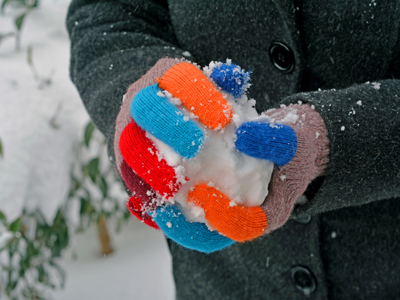The temperature is 4o. If it falls 8o it will become -4o.

# Negative Numbers 1

This Math quiz is called 'Negative Numbers 1' and it has been written by teachers to help you if you are studying the subject at elementary school. Playing educational quizzes is an enjoyable way to learn if you are in the 3rd, 4th or 5th grade - aged 8 to 11.

It costs only \$12.50 per month to play this quiz and over 3,500 others that help you with your school work. You can subscribe on the page at Join Us

Negative numbers are shown with a minus sign directly before the number. For instance -10. Negative numbers are used for measuring temperature and also to denote a loss, such as a debt. On negative number lines, the sequence of numbers goes the opposite way to that on positive number lines. For example, in this sequence: -5, -6, -7, -8... etc.

See how much you know about negative, or minus, numbers by playing this quiz.

1.
The temperature is -8o. If it rises 3o what is the temperature now?
-11o
11o
-5o
5o
-8 + 3 = -5
2.
Which of these statements is true?
-3 > 3
-1 > -7
-5 > -2
-4 > -3
The symbol > means greater than. The larger part of the symbol is next to the largest number
3.
The temperature is 4o. If it falls 8o what is the temperature now?
0o
-3o
-4o
-8o
One way to work this out is to split the 8 into 4 and 4 like this:
4 - 4 = 0
0 - 4 - = -4
4.
Which of these statements is true?
-1 < -4
-2 < -6
2 < -2
-5 < -3
The symbol < means less than. The smaller part of the symbol is next to the smallest number
5.
Which is the next number in the following sequence? 0, -1, -2, -3, -4
5
-4
-5
-6
Negative numbers go down in the same sequence that positive numbers go up in
6.
Which of these is the smallest number?
0
-1
-3
-5
Remember, the higher the negative number the lower its value
7.
Which of these is the largest number?
-1
-2
-3
-4
Negative numbers are the opposite of positive numbers therefore -1 is larger than all the other negative numbers
8.
Which are the missing numbers -1, -2, __, -4, -5, __?
-3 and -6
3 and 6
-3 and 6
3 and -6
This sequence is going down by 1 each time
9.
The temperature is -3o. If it rises 12o what is the temperature now?
9o
-9o
-15o
3o
-3 + 12 is the same as 12 - 3
10.
The temperature is -5o. If it falls 2o what is the temperature now?
7o
-3o
-7o
3o
-5 - 2= -7
Author:  Amanda Swift